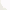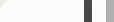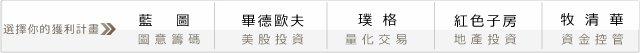## 第一次程式交易就上手－突破當日高點與低點進場策略K棒過當日低點多少次表示盤比較弱，就進場作空。#### 參數與變數設定

input : r(4),BeginTime(0900),EndTime(1245);

r是代表需要突破的次數，
BeginTime是我們開始交易的時間，
EndTime是我們終止交易的時間。

var: TH(0),TL(0),mkp(0),ax(0),ay(0),aa(0),bb(0);

TH是用來儲存當日的最高點；
TL是用來儲存當日的最低點。
mkp用來儲存我們手邊部位狀態。
ax用來計算我們作多的次數，
ay用來計算我們作空的次數。
aa用來記錄突破高點的次數，
bb用來記錄突破低點的次數。
ax跟ay可以用來限制我們當日多空交易次數。

if date <> date then begin
mkp=0;
ax=0;
ay=0;
aa=0;
bb=0;
TH=high;
TL=low;
end;

aa跟bb又要怎麼紀錄過高點跟過低點的次數呢？
if high > TH then begin
TH=high;
aa=aa+1;
end;
if low < TL then begin
TL=low;
bb=bb+1;
end;

#### 進場方式

if BeginTime < Time and Time < EndTime then begin
if MarketPosition = 0 and ax < 1 and aa > r and aa > bb then buy next bar at highd(0)+1 stop;
if MarketPosition = 0 and ay < 1 and bb > r and bb > aa then sell next bar at lowd(0)-1 stop;
end;

#### 出場方式

if marketposition =1 then begin
exitlong at entryprice-50 stop ;
if time>1339 then exitlong this bar on close;
end;
if marketposition =-1 then begin
exitshort at entryprice+50 stop ;
if time>1339 then exitshort this bar on close;
end;
setexitonclose;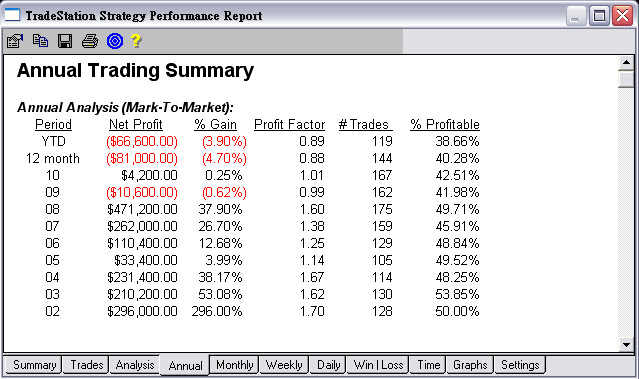1. 當你進場後，最大獲利點數小於50點的話，

2. 作多時，從最高點拉回1.5%時出場；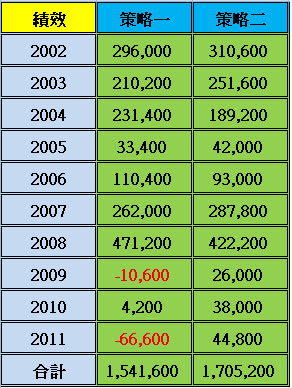DD也變小了，雖然勝率變低，因為多了保護性的出場點，

input : r(4),BeginTime(0900),EndTime(1245);
var :TH(0),TL(0),mkp(0),ax(0),ay(0),aa(0),bb(0);
if date <> date then begin
mkp=0;
ax=0;
ay=0;
aa=0;
bb=0;
TH=high;
TL=low;
end;
if high > TH then begin
TH=high;
aa=aa+1;
end;
if low < TL then begin
TL=low;
bb=bb+1;
end;
if BeginTime < Time and Time < EndTime then begin
if MarketPosition = 0 and ax < 1 and aa > r and aa > bb then buy at highd(0)+1 stop;
if MarketPosition = 0 and ay < 1 and bb > r and bb > aa then sell at lowd(0)-1 stop;
end;
mkp=marketposition;
if mkp <> 1 and mkp=1 then ax=ax+1;
if mkp <> -1 and mkp=-1 then ay=ay+1;
if marketposition =1 then begin
exitlong at entryprice-50 stop ;
if highest(h,barssinceentry)-entryprice < 50 then exitlong at lowest(low,24)-1 stop;
exitlong at highd(0)*0.985 stop;
if time>1339 then exitlong this bar on close;
end;
if marketposition =-1 then begin
exitshort at entryprice+50 stop ;
if entryprice-lowest(h,barssinceentry) < 50 then exitshort at highest(high,24)+1 stop;
exitshort at lowd(0)*1.015 stop;
if time>1339 then exitshort this bar on close;
end;
setexitonclose;

#### 0 意見: